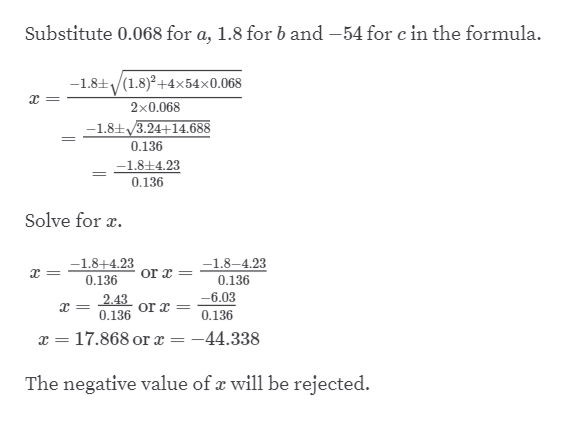# Using Social Security Administration data for selected years from 2012 and projected to 2050, the U.S. consumer price index (CPI) can be described by the equationC = 0.068  + 1.8t + 96where t is the number of years past 2010. With 2012 as the reference year, a year in which the CPI = 120.56 means goods and services that cost \$100.00 in 2012 are expected to cost \$120.56 in that year. Find the year in which the CPI is predicted to reach 150.00.

Question
152 views

Using Social Security Administration data for selected years from 2012 and projected to 2050, the U.S. consumer price index (CPI) can be described by the equation

C = 0.068  + 1.8t + 96

where t is the number of years past 2010. With 2012 as the reference year, a year in which the CPI = 120.56 means goods and services that cost \$100.00 in 2012 are expected to cost \$120.56 in that year. Find the year in which the CPI is predicted to reach 150.00.

check_circle

Step 1

Considering the Consumer price index (CPI) by the equation

C = 0.068t2  + 1.8t + 96

Step 2

This is a quadratic equation and the formula to solve is shown below:

Step 3

Applying this formula and solving for t...help_outlineImage TranscriptioncloseSubstitute 0.068 for a, 1.8 for b and -54 for c in the formula. -1.8+/(1.8)2+4x54x0.068 2x0.068 -1.8+y3.24+14.688 0.136 -1.8+4.23 0.136 Solve for . -1.8+4.23 0.136 -1.8-4.23 0.136 or x= 2.43 0,136 Or -6.03 = 0.136 17.868 or x -44.338 The negative value of a will be rejected. fullscreen

### Want to see the full answer?

See Solution

#### Want to see this answer and more?

Solutions are written by subject experts who are available 24/7. Questions are typically answered within 1 hour.*

See Solution
*Response times may vary by subject and question.
Tagged in

### Math